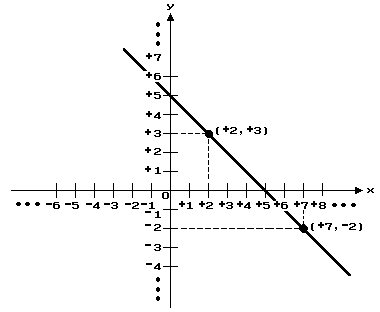College Level Math Solving a variety of algebraic equations including real numbers, exponents, radicals, polynominals, and complex numbers. Plotting points on the Cartesian plane.Graphing functions (linear, polynomial, logarithmic, and exponential) and conic sections. Converting exponential statements to logarithmic form and solving for a variable in applications. Calculating systems of equations using two and three variables by various methods, including the graphic calculator. Simplifying and evaluating expressions involving absolute value; and solving absolute value equations and inequalities.